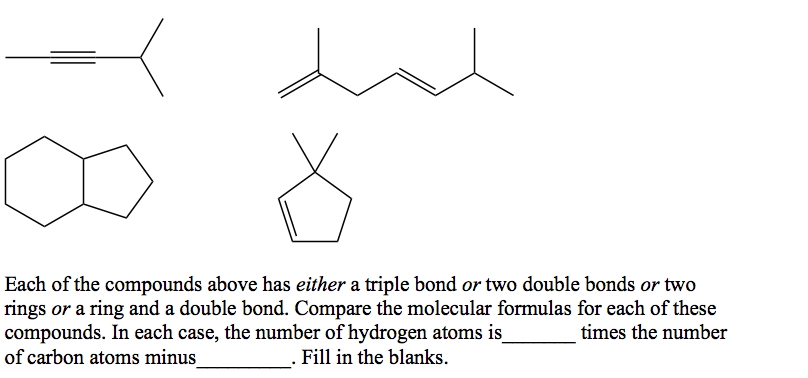# Problem: Now write out the molecular formula for each of these compounds:

91% (83 ratings)
###### Problem Details

Now write out the molecular formula for each of these compounds:What scientific concept do you need to know in order to solve this problem?

Our tutors have indicated that to solve this problem you will need to apply the Empirical Formulas concept. If you need more Empirical Formulas practice, you can also practice Empirical Formulas practice problems.

What is the difficulty of this problem?

Our tutors rated the difficulty ofNow write out the molecular formula for each of these compou...as medium difficulty.

How long does this problem take to solve?

Our expert Organic tutor, Chris took 3 minutes and 38 seconds to solve this problem. You can follow their steps in the video explanation above.

What textbook is this problem found in?

Our data indicates that this problem or a close variation was asked in Organic Chemistry - Klein 1st Edition. You can also practice Organic Chemistry - Klein 1st Edition practice problems.